# Synapse ModelThree different classes of synapse can be defined; spiking chemical, non-spiking chemical and electrical. Each class can have an unlimited number of types which differ in the quantitative values of their defining parameters. Only conductance increase chemical synapses can be simulated in this neural plug-in. The way the three classes are modeled, and the significance of the defining parameters, is described next.

### Spiking Chemical Synapses

Basic Spiking Chemical Synapse
A spiking chemical synapse is modeled as a sudden increase in post-synaptic conductance which occurs when, and only when, the pre-synaptic neuron spikes. A delay can be set between the pre-synaptic spike and the post-synaptic response. The post-synaptic conductance has a defined equilibrium (reversal) potential, and this determines whether the synapses is excitatory (equilibrium potential above spike threshold) or inhibitory (equilibrium potential below spike threshold). The conductance increase has an instantaneous onset to a defined amplitude, and then declines exponentially back to zero with a defined decay rate time constant. If a second synaptic event of this type occurs in a particular neuron before the conductance increase initiated by the first has died away, the two events sum.1. Pre-synaptic spike. 2. Delay between pre-synaptic spike and post-synaptic response. 3. Initial amplitude of post-synaptic conductance increase. 4. Decay rate (exponential time constant) of post-synaptic conductance increase.  (approximately equals time to decay to 37% of initial value).

Facilitation Model
Spiking chemical synapses can be defined as facilitating or anti-facilitating (decrementing). Facilitation is modeled as follows. If a PSP occurs immediately following a PSP of the same type from the same pre-synaptic neuron, then the amplitude of the conductance increase at the start of the second PSP is the value of the conductance increase at the start of the first PSP, multiplied by the relative facilitation factor. Thus a relative facilitation value of 1 means that no facilitation occurs, a value greater than 1 means the synaptic response increases (facilitation), while a value less than 1 means the synaptic response decreases (anti-facilitation). As the time interval between two synaptic events of the same type from the same pre-synaptic neuron increases, the degree of facilitation decays back exponentially towards a value of 1 (i.e. no facilitation) with a defined decay time constant.
NOTE: this model produces a fairly realistic qualitative simulation, but this does not imply that the model can be used to fit real data in a quantitative manner.

Paired-Pulse Facilitation
If two pre-synaptic spikes occur, the degree of facilitation depends upon the interval between them.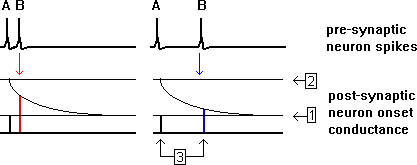1. Amplitude of initial (unfacilitated) post-synaptic conductance increase. 2. Maximally facilitated amplitude of post-synaptic conductance increase. [Relative facilitation factor = 2/1]. 3. Decay rate (exponential time constant) of facilitation. (approximately equals time to decay to 37% of initial value).

Summation of Residual Facilitation
If a series of pre-synaptic spikes occur, facilitation effects from several pre-synaptic spikes may contribute to each post-synaptic response.1. Amplitude of initial (unfacilitated) post-synaptic conductance increase. 2. Maximally facilitated amplitude of post-synaptic conductance increase. 3. Amplitude of facilitation contributed by immediately preceding pre-synaptic spike. 4. Amplitude of facilation contributed by 2nd preceding spike. 5. Amplitude of facilitation contributed by 3rd preceding spike.

Voltage-Dependent Model
Spiking chemical synapses can be defined as voltage-dependent, if desired. The underlying concept is that the post-synaptic conductance is blocked (e.g. by extracellular Mg++ ions in the case of NMDA-type channels) when the post-synaptic membrane is relatively hyperpolarised, and unblocked when the post-synaptic membrane is relatively depolarised. The mechanism is modeled as follows. First a nominal non-voltage-dependent conductance is calculated as described in the preceding sections, with a baseline initial unfacilitated level equal to the fully blocked conductance. This nominal conductance is then scaled by a factor dependent on the post-synaptic membrane potential. The scaling factor is derived from the baseline and maximum relative conductances, which define the initial values of the fully blocked and fully unblocked conductances for the unfacilitated synapse, and a threshold and saturation voltage, which define the post-synaptic voltage at which the block starts to be removed (threshold) and at which the block is fully removed (saturation).

Typical Scaling Function for a Voltage-Dependent Synapse:
Post-Synaptic Voltage -> Post-Synaptic Conductance Increase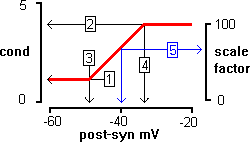1. Minimum (blocked) amplitude of initial baselin (unfacilitated) post-synaptic conductance increase. 2. Maximum (unblocked) amplitude of initial (unfacilitated) post-synaptic conductance increase. 3. Threshold post-synaptic voltage at which conductance starts to unblock. 4. Saturation post-synaptic voltage at which conductance is fully unblocked. 5. Example: at a post-synaptic membrane potential of -40 mV, the conductance will be scaled to about 75% of its nominal (non-voltage-dependent) value.

Hebbian Model
Spiking chemical synapses can be defined as having Hebbian properties, if desired. A Hebbian synapse is one in which the synaptic strength is dependent upon the degree of conjoint activity of the pre- and post-synaptic neurons. In other words, if the pre- and post-synaptic neurons are both active at relatively high frequency, the synaptic strength is augmented (“trained”). Synapses with these properties are thought to be involved in memory-related processes such as long-term potentiation (LTP) and classical conditioning.

Learning
Learning (augmentation) at Hebbian synapses is modeled as follows. Each Hebbian synaptic type has a baseline conductance which represents its naive, unaugmented state and to which all synaptic connections mediated by that type are set initially. Each neuron which receives Hebbian input retains a short-term memory (with duration set by the Hebb Time-Window parameter) in which it records the time of occurrence of any Hebbian inputs which impinge upon it. Whenever such a post-synaptic neuron spikes, it checks its short term memory to see whether a Hebbian input has occurred within the time window. If such an input has occurred, then the strength of that particular connexion is augmented. The degree of augmentation depends upon the Increment parameter, and the time interval between the input and the spike.

newG = G + Inc * (maxG - G) * ((window-interval)/ window)

where newG is the new conductance of the connexion, G is the current conductance of the connexion, Inc is the fractional increment, maxG is the maximum, fully augmented conductance, interval is the time interval between the Hebbian pre-synaptic input and the post-synaptic spike, and window is the Hebb time window.

Example of Hebbian Augmentation
 A. Spike in pre-synaptic neuron mediating Hebbian input onto post-synaptic neuron (this is the conditioned stimulus CS of classical conditioning). Left-hand vertical red line indicates post-synaptic conductance associated with left-hand EPSP (blue). B. Spike in post-synaptic neuron caused by a different pre-synaptic neuron mediating powerful supra-threshold input (this is the unconditioned stimulus US of classical conditioning). C. Second spike in pre-synaptic neuron mediating Hebbian input. Right-hand vertical red line indicates post-synaptic conductance associated with right-hand EPSP, which is augmented due to the previous conjoint occurrence of the pre-synaptic spike A and the post-synaptic spike (blue neuron) caused by input from pre-synaptic spike B.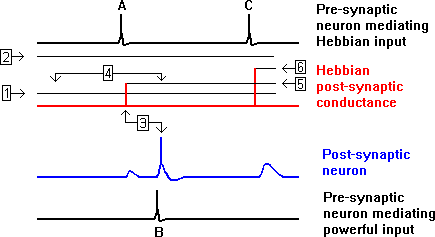1. Initial (baseline) conductance amplitude of Hebb-type synaptic connexion between pre- and post-synaptic neurons. 2. Maximum amplitude of Hebbian synapse when fully trained. Assume 15 units. 3. Time interval between Hebb-type input. from pre-synaptic spike A and post-synaptic spike B. Assume 10 msec. 4. Time-window of Hebb memory. Assume 30 msec. 5. Conductance of Hebbian synapse at time of pre-synaptic spike A. Assume 5 units. This is already partially augmented above baseline due to previous experience (not shown). 6. Further augmented conductance level of Hebbian synapse at time of pre-synaptic spike B. Assume Hebbian increment parameter is set to 0.5, then new conductance = 5 + (15-5) * 0.5 * (30-10)/30 units = 8.3 units

Forgetting
Hebbian synapses can either be set to retain augmentation indefinitely, or to “forget” unless learning is periodically enforced. Two factors control forgetting; the forgetting time window, and the consolidation factor. The two factors interact, but the former determines a baseline for how quickly synapses forget their training, and the latter determines the degree to which well trained (strongly augmented) synapses forget more slowly than weakly trained synapses.

Forgetting Time Window
If the consolidation factor is 1, then that synaptic type forgets at the same rate, irrespective of the degree of training. The augmented conductance simply declines back to baseline linearly during the forgetting time window. Thus whenever a Hebbian synaptic input occurs the post-synaptic conductance is adjusted as follows. If the time since the most recent Hebbian augmentation (i.e. a spike in the post-synaptic neuron which augmented that synapse according to the criteria described above) is greater than the forgetting window, then the synaptic conductance is set to its baseline level. If the time is within the forgetting window, then the synaptic conductance is set to a decreased level of augmentation:

newG = G - (G - baseG) * times since last Hebb event / ForgettingWindow

where baseG is the baseline conductance, ForgettingWindow is the forgetting time window, and other symbols are as defined above.

Example of Hebbian Forgetting Time Window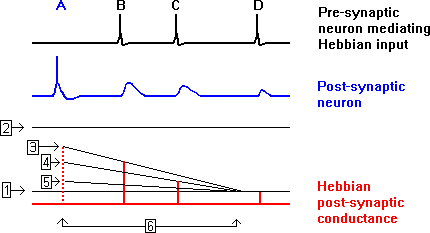A. Spike in post-synaptic neuron which augments a Hebbian synapse. The pre-synaptic spike which preceded this is not shown. This post-synaptic spike starts the clock for the forgetting time window. B. Spike in pre-synaptic neuron mediating Hebbian input occurs early in forgetting time window and elicits a conductance change which is reduced from the nominal augmented level but is still large. C. Second spike in pre-synaptic neuron mediating Hebbian input late in forgetting time window and elicits a conductance change which is reduced from actual augmented level of the previous input. D. Third spike in pre-synaptic neuron mediating Hebbian input occurs outside forgetting time window and elicits a conductance change which has returned to its baseline unaugmented level.

 1. Initial (baseline) conductance amplitude of Hebb-type synaptic connexion between pre- and post-synaptic neurons. 2. Maximum amplitude of Hebbian synapse when fully trained. 3. Nominal value of augmented conductance immediately following post-synaptic spike A which augments synapse. 4. Actual value of augmented conductance from spike B. 5. Actual value of augmented conductance from spike C. 6. Forgetting time window.

Consolidation
If the consolidation factor is greater than 1, then more strongly augmented synapses forget more slowly. For these synapses the forgetting time window is extended by product of the fractional degree of augmentation and the amount by which the consolidation factor exceeds 1.
 G% = (G - baseG) / (maxG - baseG) Inc = (consolidation - 1) * G% ForgettingWindow = baseForgettingWindow + baseForgettingWindow * Inc

where G% is the current fractional augmentation in synaptic conductance due to Hebbian learning, baseForgettingWindow is the baseline forgetting time window and other symbols are as defined above.

Example of Hebbian Consolidation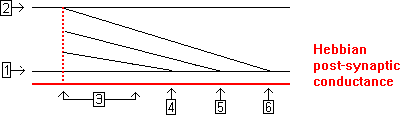1. Initial (baseline) conductance amplitude of Hebb-type synaptic connexion between pre- and post-synaptic neurons Assume 1 unit. 2. Maximal (fully augmented/trained) conductance amplitude of Hebb-type synaptic connexion between pre- and post-synaptic neurons. Assume 4 units. 3. Baseline forgetting time window. Assume 100 s Assume consolidation factor 3. 4. Forgetting time window for conductance augmented to 2 units (33%) Augmented forgetting time window = 166 s. 5. Forgetting time window for conductance augmented to 3 units (66%) Augmented forgetting time window = 233 s. 6. Forgetting time window for fully trained/augmented conductance (value 4 units, 100%) Augmented forgetting time window = 300 s.

NOTE: The quantitative model for Hebbian synapses used in this simulation is speculative and its only justification is that it produces a behaviour which approximates to what is thought may occur. However, the existence of Hebbian synapses themselves is speculativeno, and thus no real synapses have been described from which Hebbian response characteristics can be quantified.

### Non-Spiking Chemical Synapses

A non-spiking chemical synapse is modeled as a variable increase in post-synaptic conductance whose level depends upon the pre-synaptic membrane potential. The post-synaptic conductance has a defined equilibrium (reversal) potential, and this determines whether the synapses is excitatory (equilibrium potential above spike threshold) or inhibitory (equilibrium potential below spike threshold). The relationship (transfer function) between the pre-synaptic voltage and the post-synaptic conductance is determined by three paramters. The pre-synaptic voltage threshold is the minimum pre-synaptic membrane potential at which a post-synaptic conductance increase occurs; if the pre-synaptic voltage is below this value, there is no post-synaptic effect. The pre-synaptic saturation voltage is the pre-synaptic membrane potential at which the post-synaptic response reaches its maximum value; further depolarisation of the pre-synaptic neuron above this value does not increase the post-synaptic response. The maximum post-synaptic conductance amplitude is exactly that; the amplitude of the post-synaptic conductance when the pre-synaptic membrane potential reaches or exceeds the pre-synaptic saturation voltage. Between the pre-synaptic threshold and saturation voltages, the post-synaptic conductance varies linearly between 0 and the maximum amplitude.

Typical Transfer Function:
Pre-Synaptic Voltage -> Post-Synaptic Conductance Increase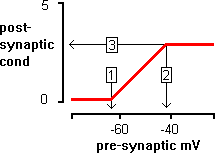1. Pre-synaptic voltage threshold. 2. Pre-synaptic saturation voltage. 3. Maximum post-synaptic conductance amplitude.

### Electrical Synapses

An electrical synapse is modeled as a non-specific electrical conductance linking two neurons. Current therefore flows from one neuron to the other whenever there is a difference between the membrane potentials of the two neurons and the junctional (electrical synapse) conductance is greater than zero. There are two types of electrical synapse:

Non-rectifying electrical synapses

Non-rectifying electrical synapses have a constant junctional conductance irrespective of the membrane potential of either neuron.

Rectifying electrical synapses
Recifying electrical synapses have a junctional conductance which varies with the voltage difference between the membrane potentials of the two neurons (junctional potential). These synapses are thus polarised, and by definition the pre-synaptic neuron is the neuron which acts as a source for positive current (i.e. depolarising potentials pass from the pre-synaptic neuron to the post-synaptic neuron, and not vice versa). The junctional potential is defined as the pre-synaptic membrane potential minus the post-synaptic membrane potential, and thus junctional potential is always positive when the rectifying synapse is in the high-conductance state.

Defining parameters
Each electrical synapse has a defined minimum conductance, which is the standard conductance of a non-rectifying synapse, or the "off" conductance of a rectifying synapse, and a defined maximum conductance, which is the "on" conducance of a rectifying synapse. For non-rectifying synapses, the maximum and minimum conductances should be set equal. Rectifying synapses have two further parameters, the turn-on junctional potential, which is the potential at which the junctional conductance starts to increase above the minimum level, and the saturation junctional potential, which is the potential at which the junctional conductance reaches maximum. Between the turn-on and saturation junctional potentials, the junctional conductance varies linearly between the minimum and maximum values.

electrical synapse: Junctional potential vs junctional conductance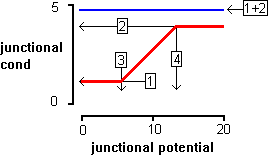Red line: A typical arbitrary rectifying electrical synapse. Blue line: A typical arbitrary non-rectifying electrical synapse.

 1. Minimum junctional conductance. 2. Maximum junctional conductance. 3. Turn-on junctional potential. 4. Saturation junctional potential.